# How to make a knight rider LED chaser (part ii)

## How to make a knight rider LED chaser (part ii)Hello guys, Welcome back to my blog. Today we are going to talk about the second or last part of the article on making a knight rider LED bulb chaser. We created one LED bulb pattern in the previous project. Do you remember it? Its code does not match a good programmer. It is a match for one LED bulb pattern, but not for multiple LED bulb patterns.

So let’s see how this code works in a few steps. I used the for loop in the Arduino language for this code.

### what is the for loop?

The loop can be described as something that happens continuously. Do you remember the void loop function? This is also the case. The for loop makes the code run again and again. See the example below.

for(int i=0 ; i <=5 ; i++);

for – For is the keyword this loop.
int i=0 – This code meaning is equal to zero.
i <=5  – Here the i value is checked. This code is used to check if five is greater than, less than, or equal. It’s called a condition
i ++  – This code increases i value one by one.

You need to remember to put a semicolon at the end of the code. This loop is run from zero to five. This loop stops when the value of i is six. Okay, let’s look at using for loop for the previous project. By studying it you can get an understanding of for loop.

Look at the code below.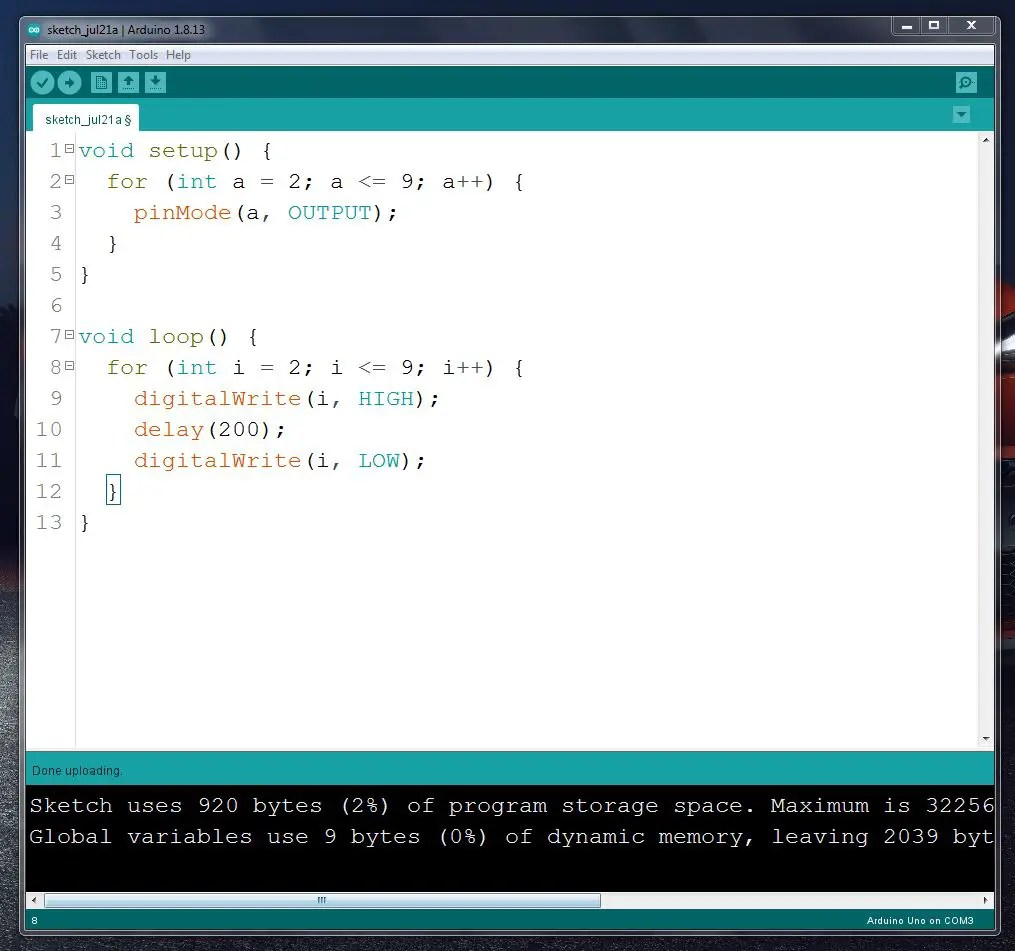void setup() {
for (int a = 2; a <= 9; a++) {
pinMode(a, OUTPUT);
}
}

void loop() {
for (int i = 2; i <= 9; i++) {
digitalWrite(i, HIGH);
delay(200);
digitalWrite(i, LOW);
}
}

The void setup includes the Arduino pins we used for this project. This loop runs from two to nine and stops when a = 10.
The code in the void loop moves the digital pins HIGH and LOW from two to nine. This loop runs from two to nine and stops when a = 10. But since this is contained in the void loop, it continues to function. So the LED bulbs blink continuously. Try this FOR LOOP for the project we did in the previous article. Please write down all your questions below. I look forward to resolving any of your issues.

So today we will see how to make multiple LED bulb patterns using Arduino basic knowledge and FOR LOOP. The above circuit has been used for this project. I have used five LED patterns for this project. Let’s do that.

Step 1
Circuit diagram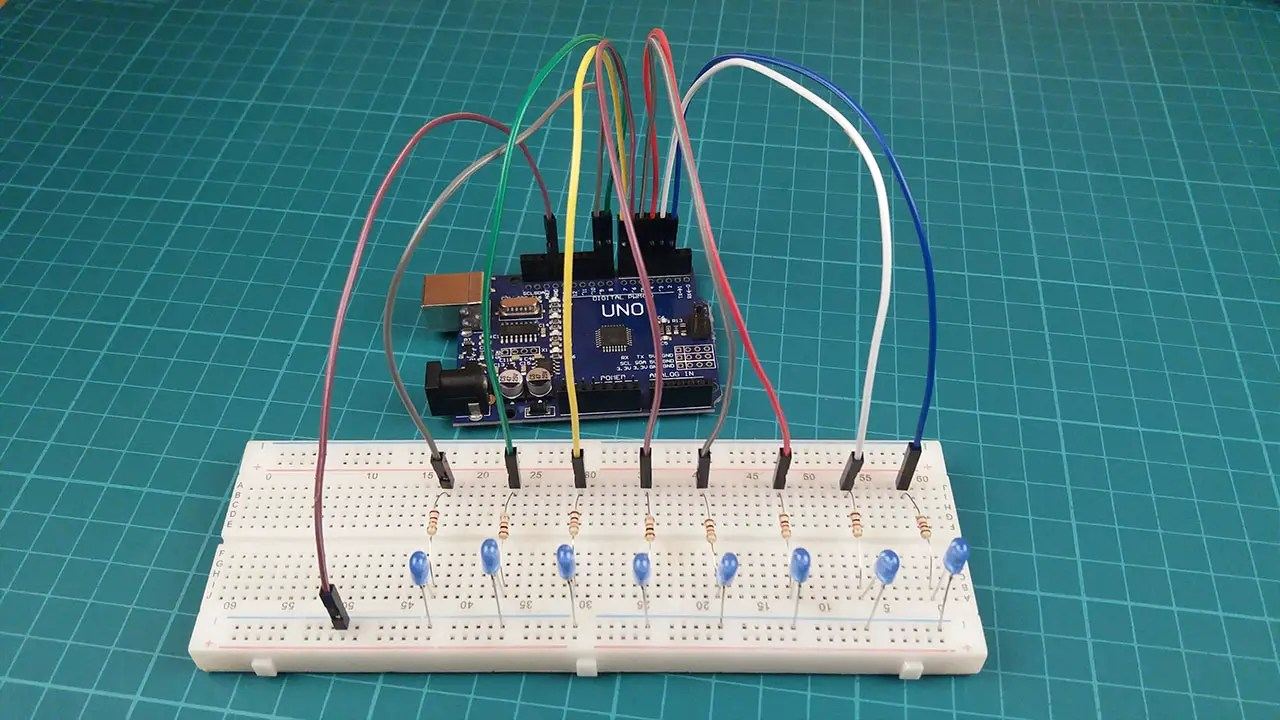How to do this circuit is described in the previous post.

Step 2
Source code

``````/*copy and paste below code */
#define t 100 //time delay
void setup() {
for (byte a = 2; a <= 9; a++) {
pinMode(a, OUTPUT);
}
}

void loop() {
//pattern one
for (byte i = 2; i <= 9; i++) {
digitalWrite(i, HIGH);
delay(t);
digitalWrite(i, LOW);
}
for (byte z = 8; z > 2; z--) {
digitalWrite(z, HIGH);
delay(t);
digitalWrite(z, LOW);
}

//pattern two
for (byte i = 2; i <= 9; i++) {
digitalWrite(i, HIGH);
digitalWrite(i + 1, HIGH);
digitalWrite(i + 2, HIGH);
delay(t);
digitalWrite(i, LOW);
digitalWrite(i - 1, LOW);
digitalWrite(i - 2, LOW);
}
for (byte z = 8; z > 2; z--) {
digitalWrite(z, HIGH);
digitalWrite(z - 1, HIGH);
digitalWrite(z - 2, HIGH);
delay(t);
digitalWrite(z, LOW);
digitalWrite(z - 1, LOW);
digitalWrite(z - 2, LOW);
}
//pattern three
for (byte i = 2; i <= 9; i++) {
digitalWrite(i, HIGH);
delay(t);
}
for (byte z = 9; z > 2; z--) {
digitalWrite(z, LOW);
delay(t);
}

//pattern four
for (byte i = 2; i <= 9; i++) {
digitalWrite(i, HIGH);
delay(t);
}
for (byte x = 2; x <= 9; x++) {
digitalWrite(x, LOW);
delay(t);
}
for (byte y = 9; y >= 2; y--) {
digitalWrite(y, HIGH);
delay(t);
}
for (byte z = 9; z >= 2; z--) {
digitalWrite(z, LOW);
delay(t);
}

//pattern five
int led  = {2, 3, 4, 5, 6, 7, 8, 9};
for (byte i = 0; i <= 8; i++) {
digitalWrite(led[i], HIGH);
}
delay(400);
for (byte i = 0; i <= 8; i++) {
digitalWrite(led[i], LOW);
}
delay(400);
}``````

Step 3
Select board and port.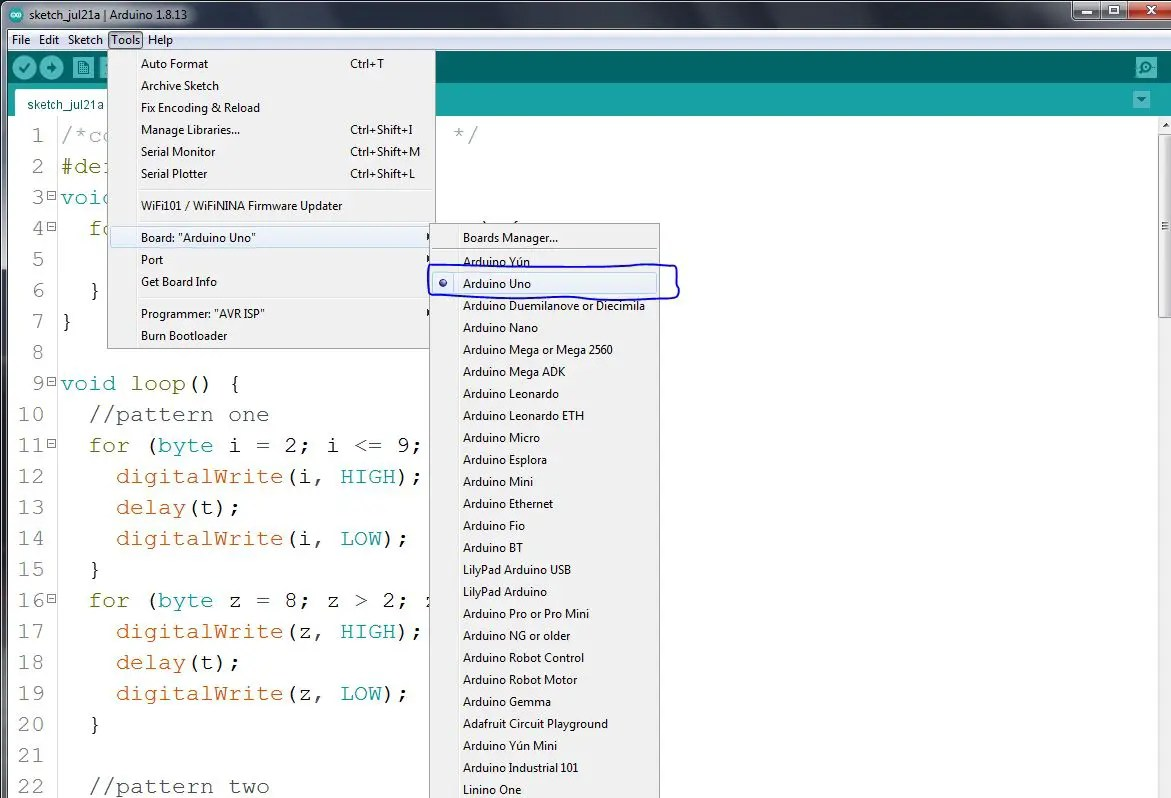Step 4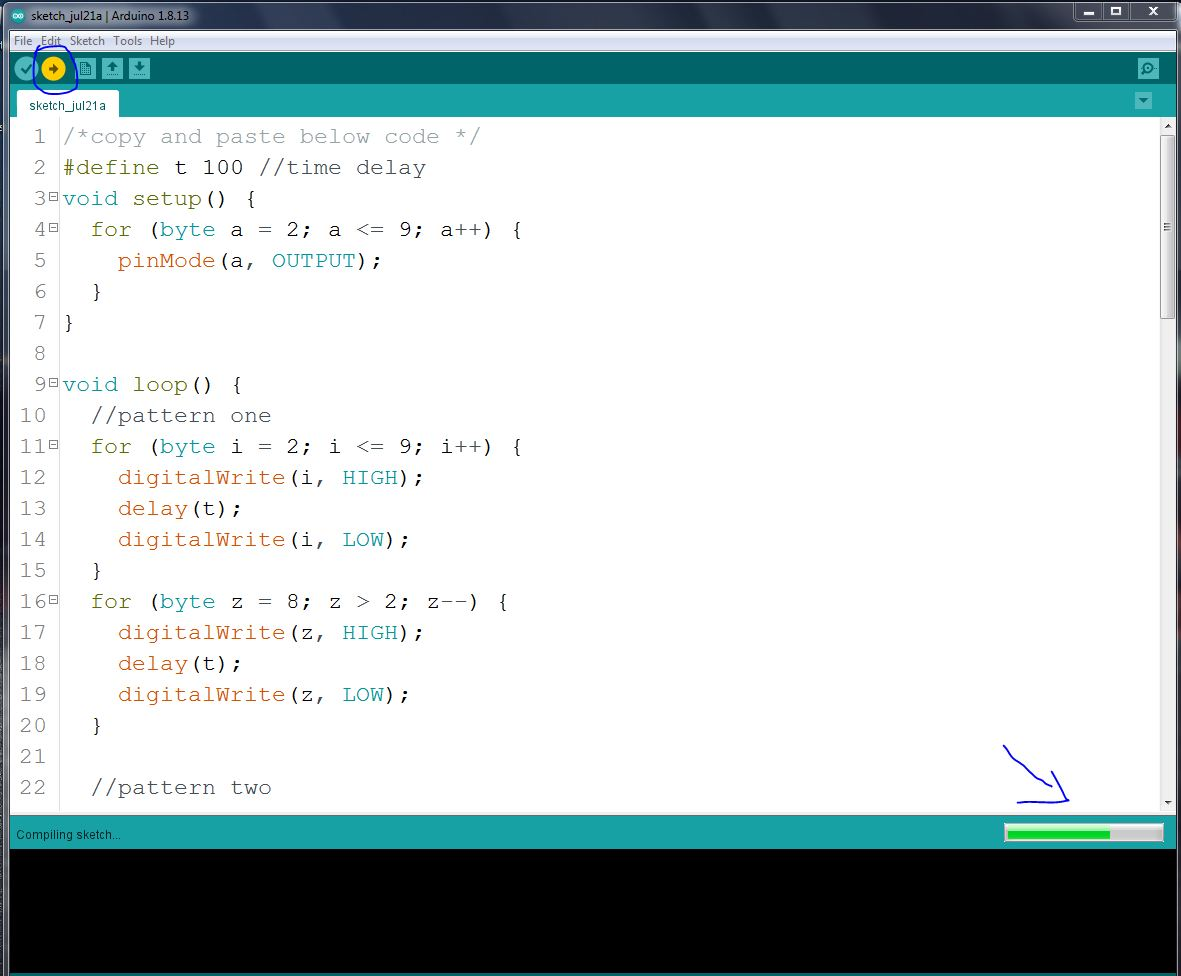This code includes five LED patterns. This is a very easy code. It was done using FOR LOOP. Please study this code and understand it. Please write down all your questions below.

The final results of this project are given below.

The following is a project using the Arduino Nano board for further study. The project includes eight LED patterns and a knight rider bulb pattern. So let’s look at it step by step.

Step 1
Circuit diagramSet up your circuit as above.

Step 2
Source code

``````/*copy and paste below code */
#define t 100
void setup() {
for (byte a = 2; a <= 13; a++) {
pinMode(a, OUTPUT);
}
}

void loop() {
Pattern1(2);
Pattern2(2);
Pattern3(2);
Pattern4(2);
Pattern5(2);
Pattern6(2);
Pattern7(2);
Pattern8(2);

}
void Pattern1(byte a) {
for (byte j = 0; j <= a; j++) {
for (byte i = 2; i <= 13; i++) {
digitalWrite(i, HIGH);
delay(t);
digitalWrite(i, LOW);
}
for (byte z = 12; z > 2; z--) {
digitalWrite(z, HIGH);
delay(t);
digitalWrite(z, LOW);
}
}

}
void Pattern2(byte a) {
for (byte j = 0; j <= a; j++) {
for (byte i = 2; i <= 13; i++) {
digitalWrite(i, HIGH);
digitalWrite(i + 1, HIGH);
digitalWrite(i + 2, HIGH);
delay(t);
digitalWrite(i, LOW);
digitalWrite(i - 1, LOW);
digitalWrite(i - 2, LOW);
}
for (byte z = 12; z > 2; z--) {
digitalWrite(z, HIGH);
digitalWrite(z - 1, HIGH);
digitalWrite(z - 2, HIGH);
delay(t);
digitalWrite(z, LOW);
digitalWrite(z - 1, LOW);
digitalWrite(z - 2, LOW);
}
}

}
void Pattern3(byte a) {
for (byte j = 0; j <= a; j++) {
for (byte i = 2; i <= 13; i++) {
digitalWrite(i, HIGH);
delay(t);
}
for (byte z = 13; z > 2; z--) {
digitalWrite(z, LOW);
delay(t);
}
}
}
void Pattern4(byte a) {
for (byte j = 0; j <= a; j++) {
for (byte i = 2; i <= 13; i++) {
digitalWrite(i, HIGH);
delay(t);
}
for (byte x = 2; x <= 13; x++) {
digitalWrite(x, LOW);
delay(t);
}
for (byte y = 13; y > 2; y--) {
digitalWrite(y, HIGH);
delay(t);
}
for (byte z = 13; z > 2; z--) {
digitalWrite(z, LOW);
delay(t);
}
}
}
void Pattern5(byte a) {
for (byte j = 0; j <= a; j++) {
int leds  = {2, 3, 4, 5, 6, 7, 8, 9, 10, 11, 12, 13};
for (byte i = 0; i <= 13; i++) {
digitalWrite(leds[i], HIGH);
}
delay(t);
for (byte i = 0; i <= 13; i++) {
digitalWrite(leds[i], LOW);
}
delay(t);
}
}
void Pattern6(byte a) {
for (byte w = 0; w <= 3; w++) {
int leds  = {2, 3, 4, 5, 6, 7, 8, 9, 10, 11, 12, 13};
for (byte i = 0; i <= 7; i++) {
digitalWrite(leds[i], HIGH);
}
delay(t);
for (byte i = 0; i <= 7; i++) {
digitalWrite(leds[i], LOW);
}
delay(t);
for (byte x = 8; x <= 13; x++) {
digitalWrite(leds[x], HIGH);
}
delay(t);
for (byte x = 8; x <= 13; x++) {
digitalWrite(leds[x], LOW);
}
delay(t);
}
}
void Pattern7(byte a) {
for (byte j = 0; j <= a; j++) {
byte w = 6;
for (byte i = 2; i <= 7; i++) {
digitalWrite(i, HIGH);
digitalWrite(i + w, HIGH);
delay(t);
digitalWrite(i, LOW);
digitalWrite(i + w, LOW);
}
}
}
void Pattern8(byte a) {
for (byte j = 0; j <= a; j++) {
byte w = 6;
for (byte i = 2; i <= 7; i++) {
digitalWrite(i, HIGH);
digitalWrite(i + w, HIGH);
delay(t);
digitalWrite(i, LOW);
digitalWrite(i + w, LOW);
}
byte u = 6;
for (byte x = 6; x > 2; x--) {
digitalWrite(x, HIGH);
digitalWrite(x + u, HIGH);
delay(t);
digitalWrite(x, LOW);
digitalWrite(x + u, LOW);
}
}
}``````

This code is also very easy. Understand by experimenting.

Step 3
verify this code.Step 4
Select board and port.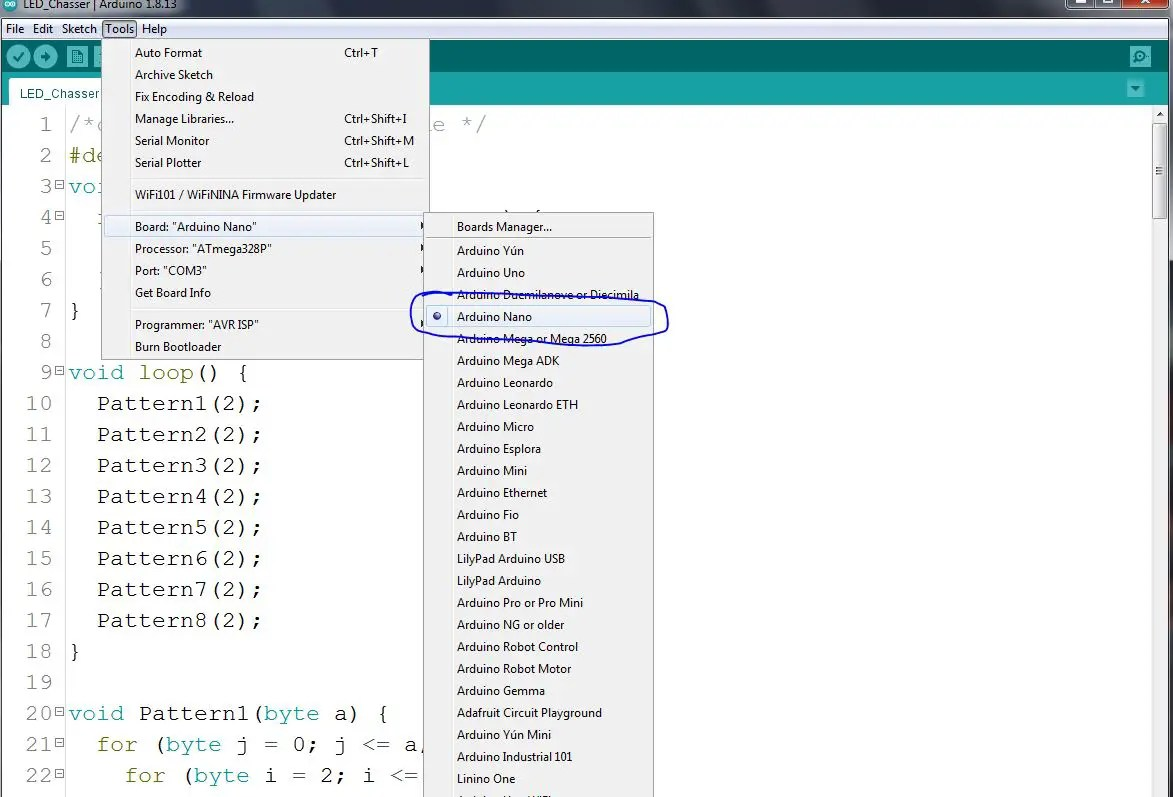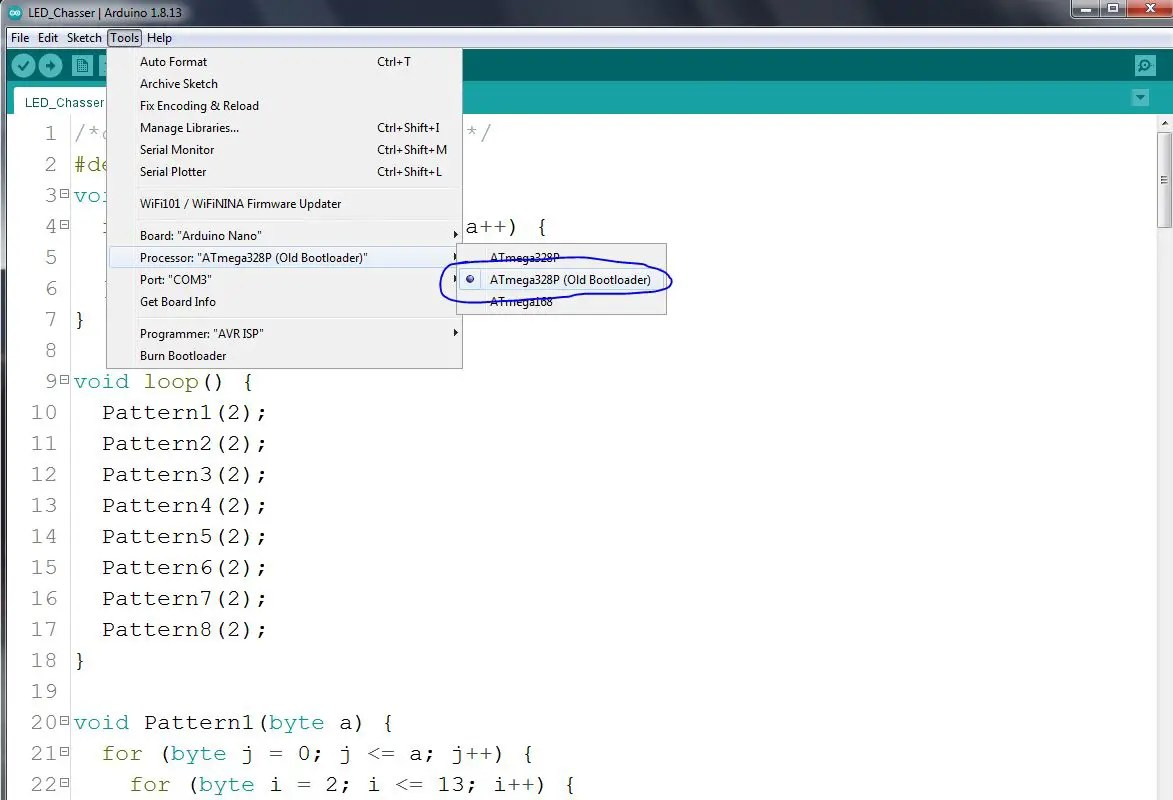Step 5